Home > ACC7 > Chapter cc310 > Lesson cc310.1.1 > Problem10-15

10-15.
1. Change each number below from a decimal to an equivalent fraction. For help with repeating decimals, review the Math Notes box from Lesson 9.2.4. Homework Help ✎

1. 0.72

2.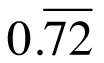3. 0.175

4.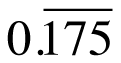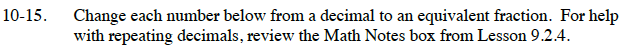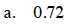How is this decimal described in words?

How do you write this as a fraction?

Reduce the fraction.

$\frac{18}{25}$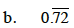By what multiple does the decimal repeat?

Multiply the decimal by its multiple (100) and subtract one set of 0.72.

Simplify.

$\frac{8}{11}$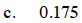See part (a).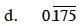See part (b).